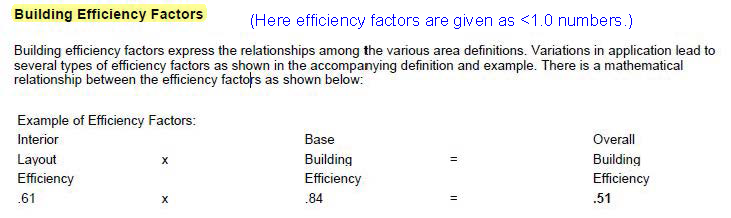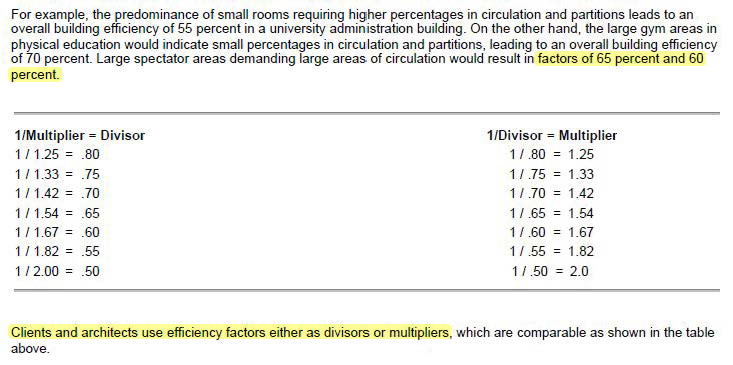•Hey Navita,

I can help with this! Since the building is estimated to cost \$500 SF, you'll first need to find out how many square feet the building needs to be reduced by to reach \$2 million in savings.

2,000,000/500 = 4,000 SF reduction

Next, subtract 4,000 SF from the GSF of the building - 108,525 GSF - 4,000 Sf = 104,525 GSF

To meet the budget, the building can't be over 104,525 GSF. Since the NSF of the building is not changing (72,350 NSF), you'll need to figure out which efficiency factor meets this requirement.

104,525 GSF/72,350 NSF = 1.44

Hope this helps!

•Thank you, Nick!

It makes sense now.

Could you also please let me know the answer this question from the same case study-

"What is the minimum number of off-street parking spaces required for the new Event Center? "

•Question:

All of our study materials define "efficiency ratio" or factor as "net to gross".  (below 1.0)

The answer to this question is "gross to net".  Is that not a "grossing factor" rather than an "efficiency factor"?  (above 1.0)

•(Edited )

Hey Robin,

You're right, efficiency ratios are under 1.0 and are typically expressed as a percentage. The example from the demo exam does not express this value as a ratio or even call it a ratio. The efficiency ratio of the above item would be 71% assuming an efficiency factor (grossing factor or space factor) of 1.4. If you navigate to page 4 of the Program Elements resource within the case study, you will see that the current building is designed with a efficiency factor of 1.5.

•Thank you, Nick.  I guess what is confusing me is that there are discrepancies of terminology.   I would like to nail this down in case I come across a similar problem in the future that is a “fill-in-the-blank” instead of a multiple choice.  The multiple choice answers to this problem indicate that we are talking about a >1.0 answer, but not all questions are multiple choice.

“Problem Seeking” uses the term “Building Efficiency Factor” with respect to <1.0 numbers (see below)  ....  but <1.0 numbers are instead customarily used with the term “Building Efficiency”.  Then below that they use the term “factor” in discussing percents.

The reason this is important in a fill-in-the-blank question is that we need to be able to determine whether we are being asked for NET/GROSS or GROSS/NET.

I understand the math involved in this question, but the terminology seems very fuzzy to me.  In researching online it gets even more confusing.  As you stated, “space factor” is used, but there are also “load factor” and “grossing factor”.  They seem to be fairly interchangeable, but the bottom line is that we need to know exactly what is being asked and since “Problem Seeking” is one of the main study resources, I would like to request some further clarification, as one answer is the inverse of the other.  (Am also assuming that this could possibly come up in PPD, as I have passed PA.)

Are we supposed to assume that the >1.0 answer (GROSS/NET) is the one requested merely because of the terminology in the bottom line of the last page of the Program Elements ... or because the multiple choice answers are in that format ... and what if it would be a fill-in-the-blank question instead?

From the book:We would typically describe this .51 efficiency as 51% efficiency and it represents NET/GROSS, right?  But here they are using the same terminology ("building efficiency factor") as the demo exam question which is requesting a number >1.0 (GROSS/NET), so how are we to know what is requested?

Again from the book:Thank you.

•(Edited )

Hey Robin,

What I can tell you is if you get a question on this topic, there will be information within the item or case study that assists you in making this determination. In the original example, the case study program resource used an efficiency factor of 1.5. That information is critical when determining the correct answer of 1.4.

One thing to remember, when an ARE item is authored, there are many architects that must approve the item prior to it making it onto the ARE. All architects must agree that the terminology and language is correct. If there is disagreement between the architects on terminology, they typically include clarifying or additional information to ensure candidates are not confused on how to answer the question. This applies to all items that contain terminology that isn't consistent across the architectural profession.

Another example of this would be vapor retarders/barriers. There is great debate on this topic, specifically within the transition zone of the US. Similar to building efficiency factor/ratio/percentage. etc., we would include information that cues in candidates on how to respond to the question.

Based on your research and understanding of this content, you are going to be fine on the exam.

•Thank you, Nick. Good to know.